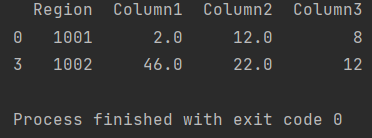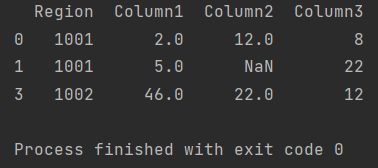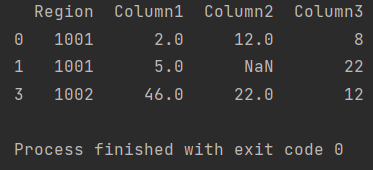Pandas How To Uncategorized How to query a dataframe

# How to query a dataframe

In this post you will learn how to query a dataframe in Pandas.

Pandas includes a query function that offers many different possibilities. I want to share some different possibilities of the query function.

## How to query a column

We’ll start with the simplest case. The example below shows how to apply a query to a column in Pandas. I would like to display only values that are greater than the number I entered.

```import pandas as pd

my_df = pd.DataFrame({'Region': ['1001', '1001', '1002', '1002'],
'Column1': [2, 5, None, 46],
'Column2': [12, None, 5, 22],
'Column3': [8, 22, 9, 12]})

my_df_11 = my_df.query('Column2>11')
print(my_df_11)

```As a parameter of the query function, I put the greater than sign in quotation marks.

## How to query a column with not

In this case, I will show how to use the query function for columns that do not contain the given value.

```import pandas as pd

my_df = pd.DataFrame({'Region': ['1001', '1001', '1002', '1002'],
'Column1': [2, 5, None, 46],
'Column2': [12, None, 5, 22],
'Column3': [8, 22, 9, 12]})

my_df_not_5 = my_df.query('not (Column2==5)')
print(my_df_not_5)
```Otherwise, you can also write it like this.

```my_df_not_5 = my_df.query("Column2 != 5")
```

## How to query a column with simple math

In the query method, you can enter simple math operations.

```import pandas as pd

my_df = pd.DataFrame({'Region': ['1001', '1001', '1002', '1002'],
'Column1': [2, 5, None, 46],
'Column2': [12, None, 5, 22],
'Column3': [8, 22, 9, 12]})

my_df_sqrt = my_df.query("sqrt(Column2) > 3")
print(my_df_sqrt)
```These are some examples of using the query method in Pandas.

Documentation of query function
How to calculate cumulative sum in Pandas
How to replace nan by mean

Tags:

## How to fix TypeError: got an unexpected keyword argumentHow to fix TypeError: got an unexpected keyword argument

You came here because you encountered a TypeError: got an unexpected keyword argument error in Pandas.

## How to fix AttributeError: module ‘pandas’ has no attribute ‘DataFrame’How to fix AttributeError: module ‘pandas’ has no attribute ‘DataFrame’

In this post I am showing you how to fix AttributeError: module ‘pandas’ has no attribute ‘DataFrame’ in Pandas.